# RD Sharma Solutions for Class 8 Maths Chapter 14 Compound Interest Exercise 14.4

In our day-to-day life, we see that there are entities like the population of a city, the value of property etc which increase in magnitude over a period of time. In Exercise 14.4 of Chapter 14, we shall see how the formulae for computing amount and compound interest are used to calculate the growth of population etc. RD Sharma Solutions can be utilised by students for detailed solutions to the problems. The solutions are made available in the pdf format where students can download easily from the links given below and start preparing for their exams. On regular practice, students develop a good amount of knowledge over the subject.

## Download the Pdf of RD Sharma Solutions for Class 8 Maths Exercise 14.4 Chapter 14 Compound Interest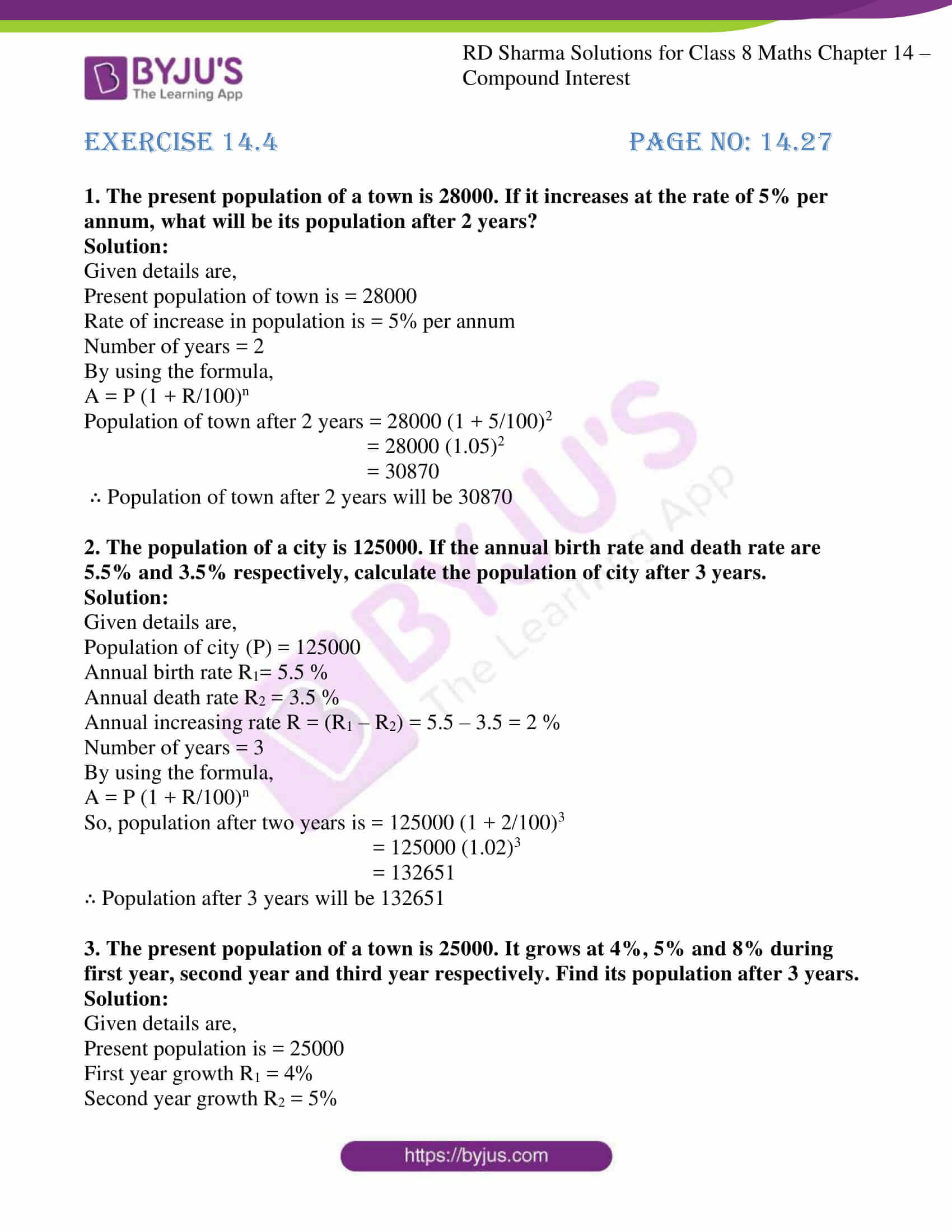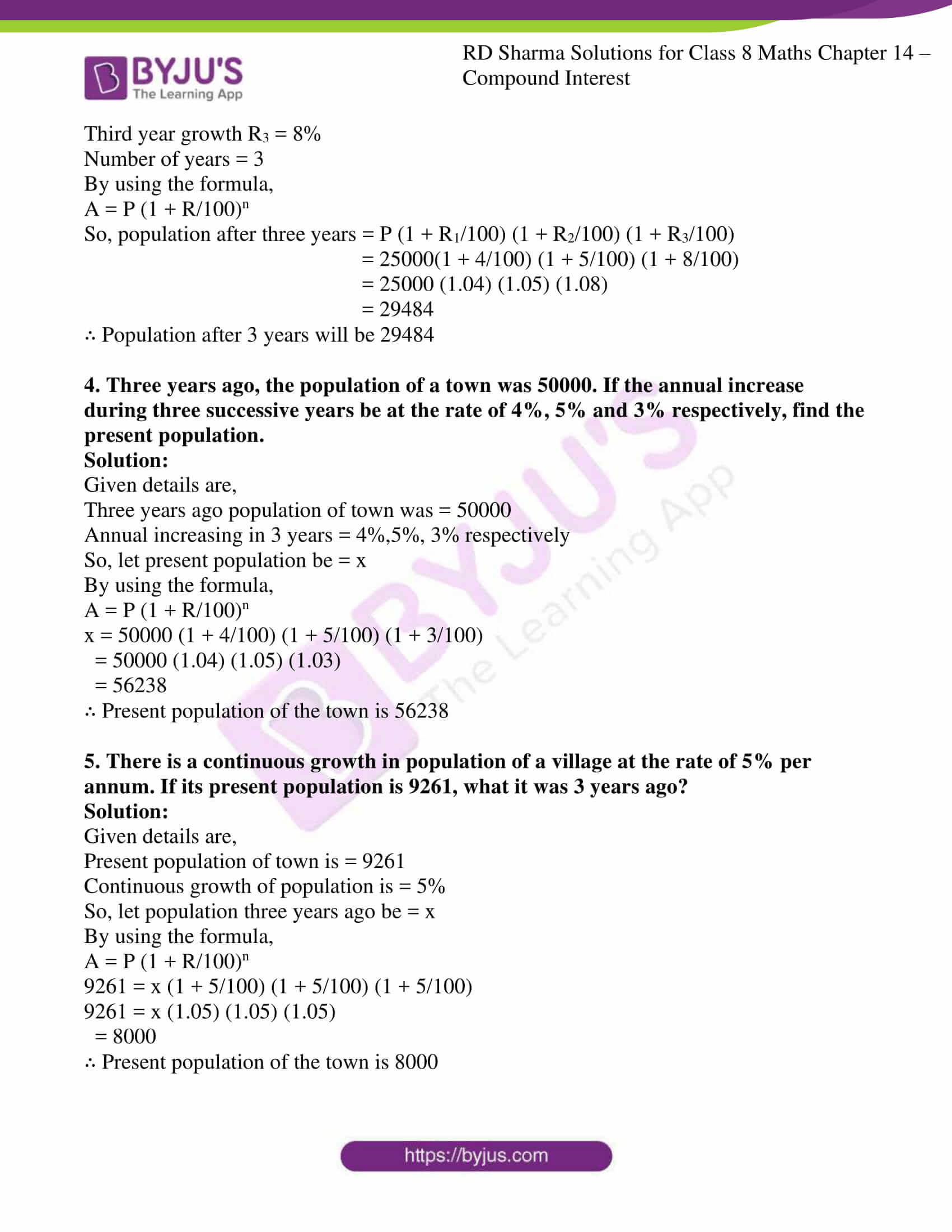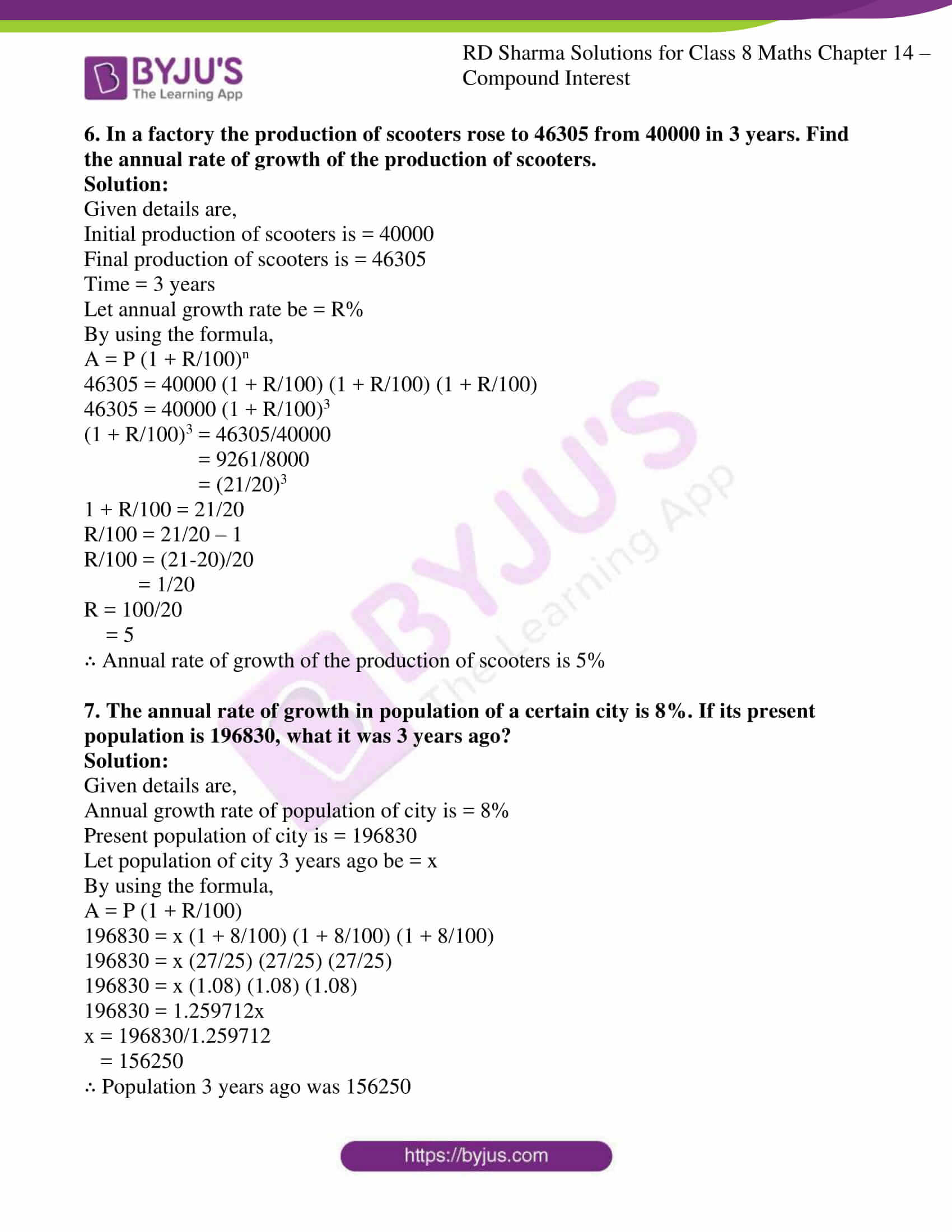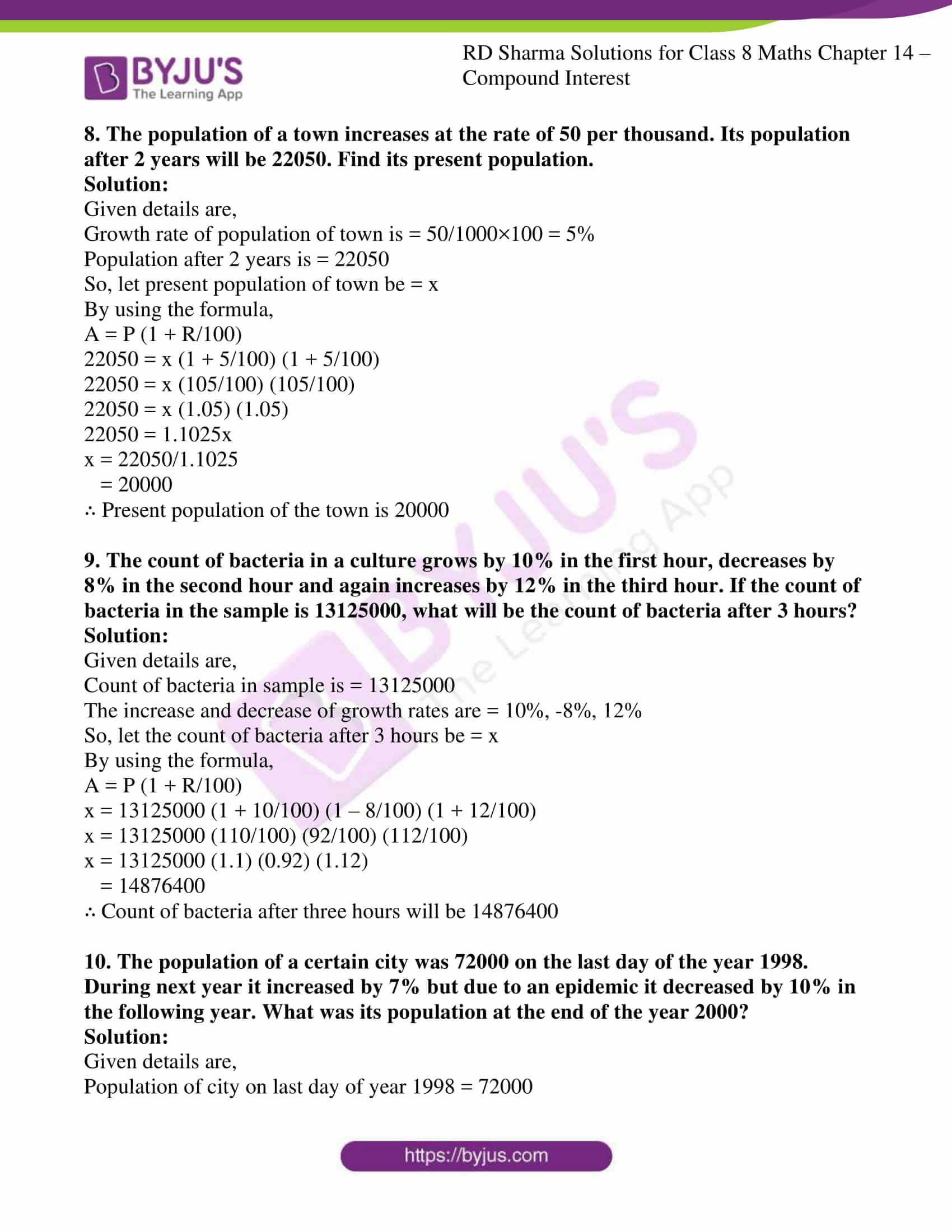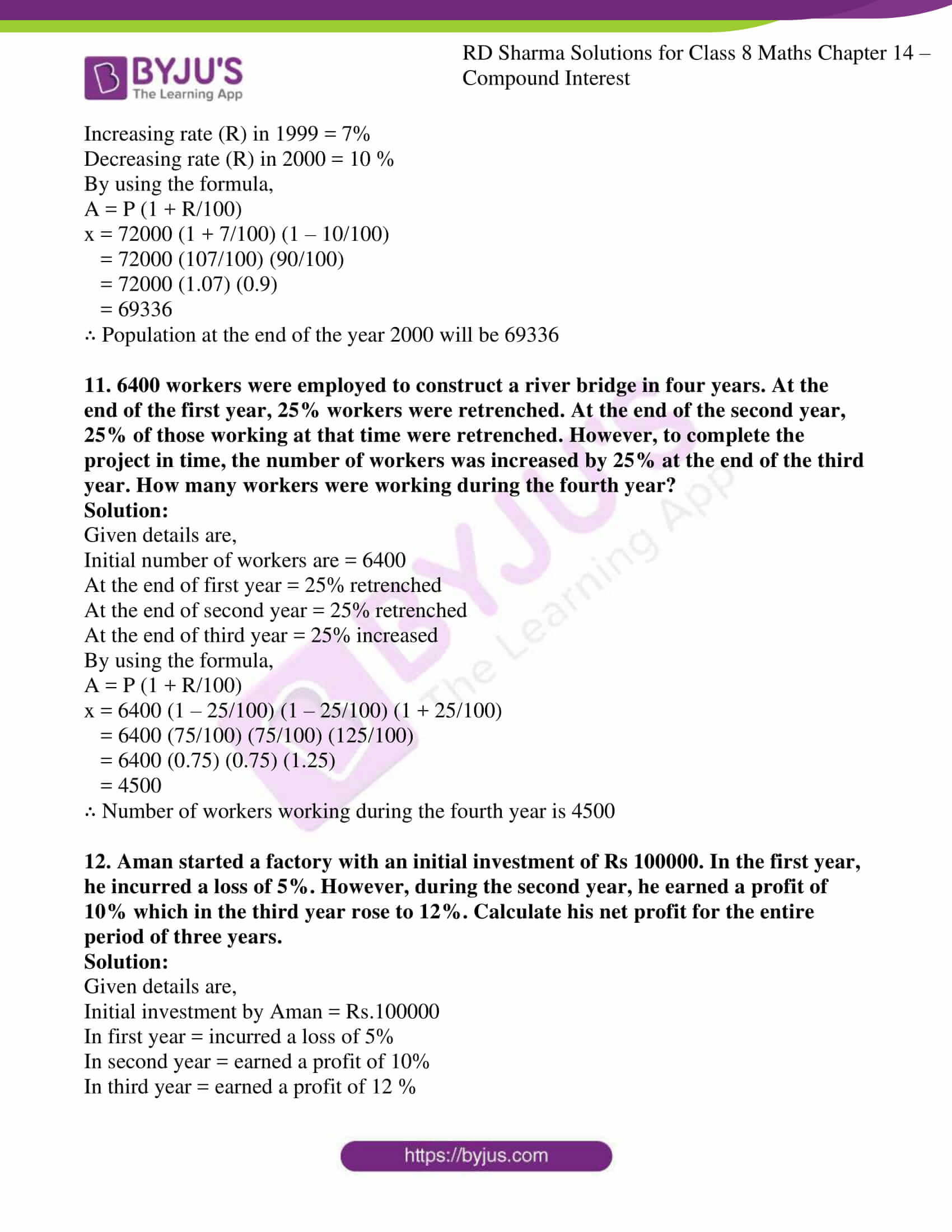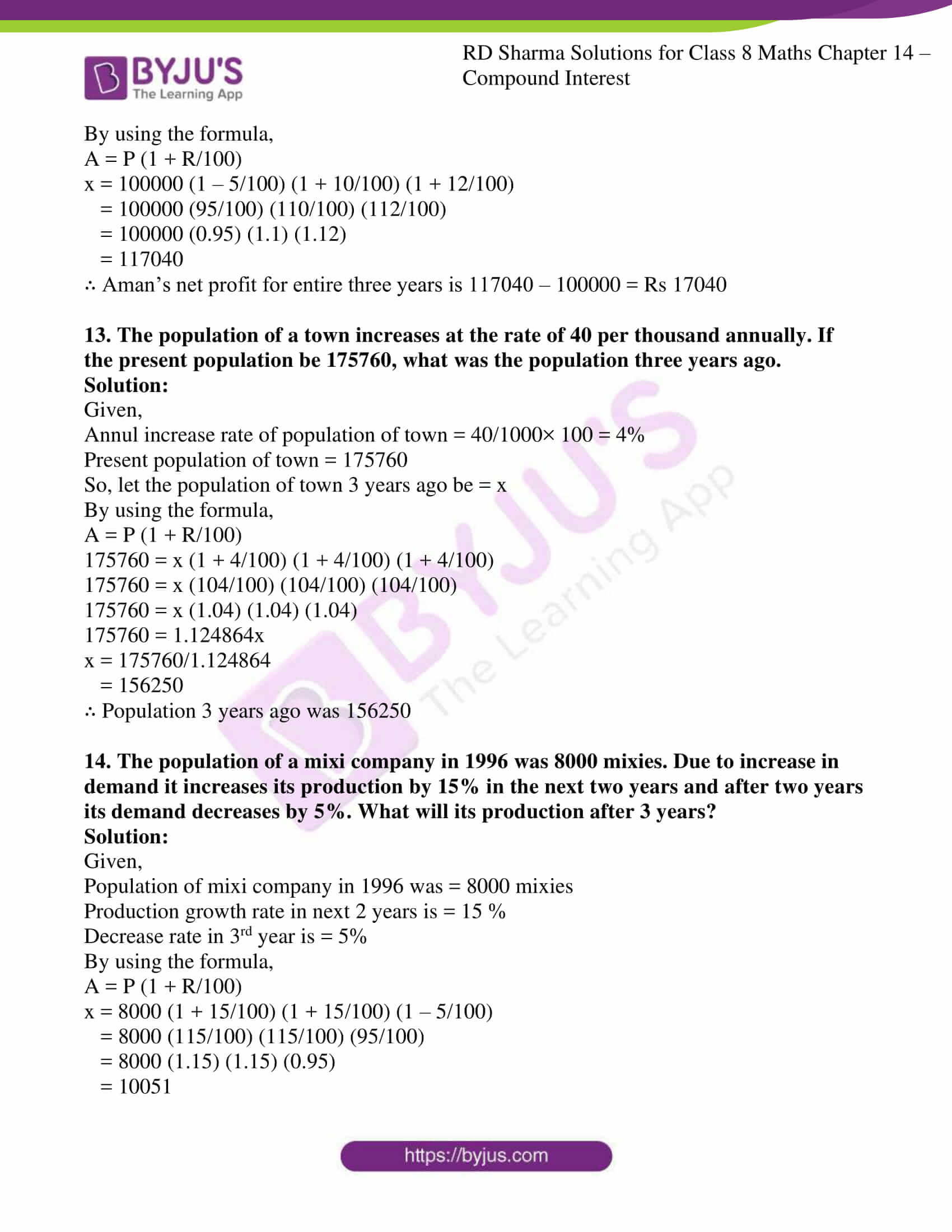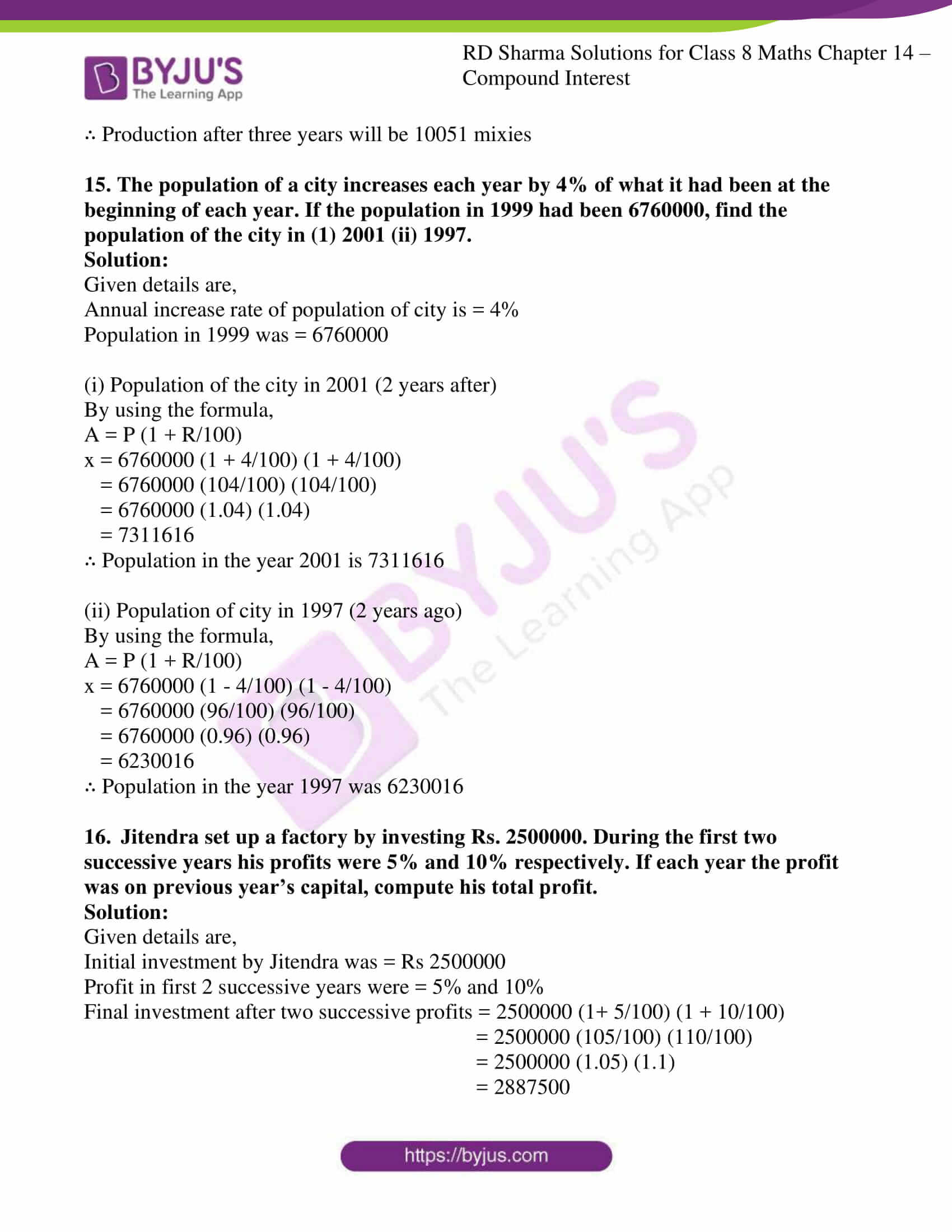### Access answers to Maths RD Sharma Solutions For Class 8 Exercise 14.4 Chapter 14 Compound Interest

1. The present population of a town is 28000. If it increases at the rate of 5% per annum, what will be its population after 2 years?

Solution:

Given details are,

Present population of town is = 28000

Rate of increase in population is = 5% per annum

Number of years = 2

By using the formula,

A = P (1 + R/100)n

Population of town after 2 years = 28000 (1 + 5/100)2

= 28000 (1.05)2

= 30870

∴ Population of town after 2 years will be 30870

2. The population of a city is 125000. If the annual birth rate and death rate are 5.5% and 3.5% respectively, calculate the population of city after 3 years.

Solution:

Given details are,

Population of city (P) = 125000

Annual birth rate R1= 5.5 %

Annual death rate R2 = 3.5 %

Annual increasing rate R = (R1 – R2) = 5.5 – 3.5 = 2 %

Number of years = 3

By using the formula,

A = P (1 + R/100)n

So, population after two years is = 125000 (1 + 2/100)3

= 125000 (1.02)3

= 132651

∴ Population after 3 years will be 132651

3. The present population of a town is 25000. It grows at 4%, 5% and 8% during first year, second year and third year respectively. Find its population after 3 years.

Solution:

Given details are,

Present population is = 25000

First year growth R1 = 4%

Second year growth R2 = 5%

Third year growth R3 = 8%

Number of years = 3

By using the formula,

A = P (1 + R/100)n

So, population after three years = P (1 + R1/100) (1 + R2/100) (1 + R3/100)

= 25000(1 + 4/100) (1 + 5/100) (1 + 8/100)

= 25000 (1.04) (1.05) (1.08)

= 29484

∴ Population after 3 years will be 29484

4. Three years ago, the population of a town was 50000. If the annual increase during three successive years be at the rate of 4%, 5% and 3% respectively, find the present population.

Solution:

Given details are,

Three years ago population of town was = 50000

Annual increasing in 3 years = 4%,5%, 3% respectively

So, let present population be = x

By using the formula,

A = P (1 + R/100)n

x = 50000 (1 + 4/100) (1 + 5/100) (1 + 3/100)

= 50000 (1.04) (1.05) (1.03)

= 56238

∴ Present population of the town is 56238

5. There is a continuous growth in population of a village at the rate of 5% per annum. If its present population is 9261, what it was 3 years ago?

Solution:

Given details are,

Present population of town is = 9261

Continuous growth of population is = 5%

So, let population three years ago be = x

By using the formula,

A = P (1 + R/100)n

9261 = x (1 + 5/100) (1 + 5/100) (1 + 5/100)

9261 = x (1.05) (1.05) (1.05)

= 8000

∴ Present population of the town is 8000

6. In a factory the production of scooters rose to 46305 from 40000 in 3 years. Find the annual rate of growth of the production of scooters.

Solution:

Given details are,

Initial production of scooters is = 40000

Final production of scooters is = 46305

Time = 3 years

Let annual growth rate be = R%

By using the formula,

A = P (1 + R/100)n

46305 = 40000 (1 + R/100) (1 + R/100) (1 + R/100)

46305 = 40000 (1 + R/100)3

(1 + R/100)3 = 46305/40000

= 9261/8000

= (21/20)3

1 + R/100 = 21/20

R/100 = 21/20 – 1

R/100 = (21-20)/20

= 1/20

R = 100/20

= 5

∴ Annual rate of growth of the production of scooters is 5%

7. The annual rate of growth in population of a certain city is 8%. If its present population is 196830, what it was 3 years ago?

Solution:

Given details are,

Annual growth rate of population of city is = 8%

Present population of city is = 196830

Let population of city 3 years ago be = x

By using the formula,

A = P (1 + R/100)

196830 = x (1 + 8/100) (1 + 8/100) (1 + 8/100)

196830 = x (27/25) (27/25) (27/25)

196830 = x (1.08) (1.08) (1.08)

196830 = 1.259712x

x = 196830/1.259712

= 156250

∴ Population 3 years ago was 156250

8. The population of a town increases at the rate of 50 per thousand. Its population after 2 years will be 22050. Find its present population.

Solution:

Given details are,

Growth rate of population of town is = 50/1000×100 = 5%

Population after 2 years is = 22050

So, let present population of town be = x

By using the formula,

A = P (1 + R/100)

22050 = x (1 + 5/100) (1 + 5/100)

22050 = x (105/100) (105/100)

22050 = x (1.05) (1.05)

22050 = 1.1025x

x = 22050/1.1025

= 20000

∴ Present population of the town is 20000

9. The count of bacteria in a culture grows by 10% in the first hour, decreases by 8% in the second hour and again increases by 12% in the third hour. If the count of bacteria in the sample is 13125000, what will be the count of bacteria after 3 hours?

Solution:

Given details are,

Count of bacteria in sample is = 13125000

The increase and decrease of growth rates are = 10%, -8%, 12%

So, let the count of bacteria after 3 hours be = x

By using the formula,

A = P (1 + R/100)

x = 13125000 (1 + 10/100) (1 – 8/100) (1 + 12/100)

x = 13125000 (110/100) (92/100) (112/100)

x = 13125000 (1.1) (0.92) (1.12)

= 14876400

∴ Count of bacteria after three hours will be 14876400

10. The population of a certain city was 72000 on the last day of the year 1998. During next year it increased by 7% but due to an epidemic it decreased by 10% in the following year. What was its population at the end of the year 2000?

Solution:

Given details are,

Population of city on last day of year 1998 = 72000

Increasing rate (R) in 1999 = 7%

Decreasing rate (R) in 2000 = 10 %

By using the formula,

A = P (1 + R/100)

x = 72000 (1 + 7/100) (1 – 10/100)

= 72000 (107/100) (90/100)

= 72000 (1.07) (0.9)

= 69336

∴ Population at the end of the year 2000 will be 69336

11. 6400 workers were employed to construct a river bridge in four years. At the end of the first year, 25% workers were retrenched. At the end of the second year, 25% of those working at that time were retrenched. However, to complete the project in time, the number of workers was increased by 25% at the end of the third year. How many workers were working during the fourth year?

Solution:

Given details are,

Initial number of workers are = 6400

At the end of first year = 25% retrenched

At the end of second year = 25% retrenched

At the end of third year = 25% increased

By using the formula,

A = P (1 + R/100)

x = 6400 (1 – 25/100) (1 – 25/100) (1 + 25/100)

= 6400 (75/100) (75/100) (125/100)

= 6400 (0.75) (0.75) (1.25)

= 4500

∴ Number of workers working during the fourth year is 4500

12. Aman started a factory with an initial investment of Rs 100000. In the first year, he incurred a loss of 5%. However, during the second year, he earned a profit of 10% which in the third year rose to 12%. Calculate his net profit for the entire period of three years.

Solution:

Given details are,

Initial investment by Aman = Rs.100000

In first year = incurred a loss of 5%

In second year = earned a profit of 10%

In third year = earned a profit of 12 %

By using the formula,

A = P (1 + R/100)

x = 100000 (1 – 5/100) (1 + 10/100) (1 + 12/100)

= 100000 (95/100) (110/100) (112/100)

= 100000 (0.95) (1.1) (1.12)

= 117040

∴ Aman’s net profit for entire three years is 117040 – 100000 = Rs 17040

13. The population of a town increases at the rate of 40 per thousand annually. If the present population be 175760, what was the population three years ago.

Solution:

Given,

Annul increase rate of population of town = 40/1000× 100 = 4%

Present population of town = 175760

So, let the population of town 3 years ago be = x

By using the formula,

A = P (1 + R/100)

175760 = x (1 + 4/100) (1 + 4/100) (1 + 4/100)

175760 = x (104/100) (104/100) (104/100)

175760 = x (1.04) (1.04) (1.04)

175760 = 1.124864x

x = 175760/1.124864

= 156250

∴ Population 3 years ago was 156250

14. The population of a mixi company in 1996 was 8000 mixies. Due to increase in demand it increases its production by 15% in the next two years and after two years its demand decreases by 5%. What will its production after 3 years?

Solution:

Given,

Population of mixi company in 1996 was = 8000 mixies

Production growth rate in next 2 years is = 15 %

Decrease rate in 3rd year is = 5%

By using the formula,

A = P (1 + R/100)

x = 8000 (1 + 15/100) (1 + 15/100) (1 – 5/100)

= 8000 (115/100) (115/100) (95/100)

= 8000 (1.15) (1.15) (0.95)

= 10051

∴ Production after three years will be 10051 mixies

15. The population of a city increases each year by 4% of what it had been at the beginning of each year. If the population in 1999 had been 6760000, find the population of the city in (1) 2001 (ii) 1997.

Solution:

Given details are,

Annual increase rate of population of city is = 4%

Population in 1999 was = 6760000

(i) Population of the city in 2001 (2 years after)

By using the formula,

A = P (1 + R/100)

x = 6760000 (1 + 4/100) (1 + 4/100)

= 6760000 (104/100) (104/100)

= 6760000 (1.04) (1.04)

= 7311616

∴ Population in the year 2001 is 7311616

(ii) Population of city in 1997 (2 years ago)

By using the formula,

A = P (1 + R/100)

x = 6760000 (1 – 4/100) (1 – 4/100)

= 6760000 (96/100) (96/100)

= 6760000 (0.96) (0.96)

= 6230016

∴ Population in the year 1997 was 6230016

16. Jitendra set up a factory by investing Rs. 2500000. During the first two successive years his profits were 5% and 10% respectively. If each year the profit was on previous year’s capital, compute his total profit.

Solution:

Given details are,

Initial investment by Jitendra was = Rs 2500000

Profit in first 2 successive years were = 5% and 10%

Final investment after two successive profits = 2500000 (1+ 5/100) (1 + 10/100)

= 2500000 (105/100) (110/100)

= 2500000 (1.05) (1.1)

= 2887500

∴ Jitendra total profit is = 2887500 – 2500000 = Rs 387500